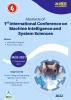# Artificial Neural Network

## Authors

Pallabi Sarkar
Techno College of Engineering Agartala, Maheskhala, Tripura
Sankha Subhra Debnath
Techno College of Engineering Agartala, Maheskhala, Tripura

### Synopsis

Background: Artificial Neural Networks is inspired by the human brain it’s can be used for machine learning and artificial neural network is to some extent modeled on the structure of the biological brain. An artificial neural network is provided by the inventor of one of the first neuron computers, Dr. Robert Hecht Nielsen the artificial neural networks is a function imitation of simplified modal of the biological neurons and their goal is to construct useful computers for real world problems.

Objectives: Artificial neural network not magic, but design based on solid mathematical methods. Neural networks better than computer for processing of sensorial data such as signal processing of sensorial data such as signal processing, image processing, pattern recognition, robot control, non-linear modeling and prediction. Practical approach for using artificial neural networks in various technical, organizational and economic applications.

Methodology:  Artificial neural networks consist of a large number of independent computational units that are able to influence the computations of each other. A neural has several inputs, and one output. The output from a neuron is calculated as a function   of a weighted sum of the inputs several neurons can be connected thus forming a neural network. simple neural networks of the kind used here (feed forward networks) are closely related to the weight matrix method (von Heijne1986) the two main difference being that the weights in neural networks are found by training rather that statistical analysis, and that neural networks are able to solve non-linear classification problem by introducing a layer of” hidden neurons” between input and output.

Result and discussion: The structure of the neural networks to compare different algorithms of neural networks training the two algorithms of trainer and trainable have been used. In each algorithm, seven   activating functions have been used.

Future work: Composition of music hand written documents to be automatically transformed into formatted word processing documents.Published
January 28, 2022